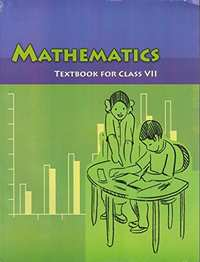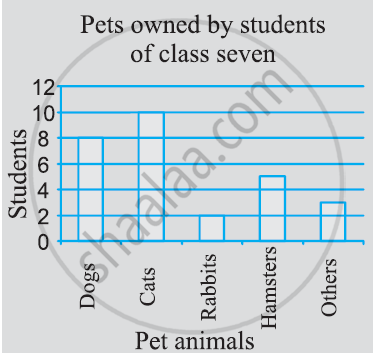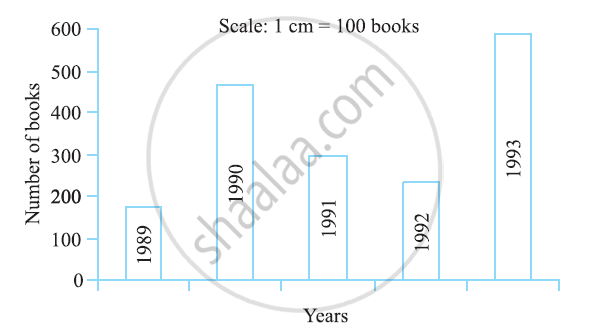Share

# NCERT solutions for Class 7 Mathematics Textbook chapter 3 - Data Handling [Latest edition]

Course
Textbook page

#### Chapters## Chapter 3: Data Handling

Ex. 3.1Ex. 3.2Ex. 3.3Ex. 3.4

#### NCERT solutions for Class 7 Mathematics Textbook Chapter 3 Data Handling Exercise 3.1 [Pages 62 - 63]

Ex. 3.1 | Q 1 | Page 62

Find the range of heights of any ten students of your class.

Ex. 3.1 | Q 2 | Page 62

Organise the following marks in a class assessment, in a tabular form.

4, 6, 7, 5, 3, 5, 4, 5, 2, 6, 2, 5, 1, 9, 6, 5, 8, 4, 6, 7

1) Which number is the highest?

2) Which number is the lowest?

3) What is the range of the data?

4) Find the arithmetic mean.

4) What is the range of the data?

Ex. 3.1 | Q 3 | Page 62

Find the mean of the first five whole numbers.

Ex. 3.1 | Q 4 | Page 62

A cricketer scores the following runs in eight innings:

58, 76, 40, 35, 46, 45, 0, 100

Find the mean score.

Ex. 3.1 | Q 5 | Page 63

Following table shows the points of each player scored in four games:

 Player Game 1 Game 2 Game 3 Game 4 A 14 16 10 10 B 0 8 6 4 C 8 11 Did not play 13

1) Find the mean to determine A’s average number of points scored per game

2)  To find the mean number of points per game for C, would you divide the total points by 3 or by 4? Why?

3) B played in all the four games. How would you find the mean?

4) Who is the best performer?

Ex. 3.1 | Q 6 | Page 63

The marks (out of 100) obtained by a group of students in a science test are 85, 76, 90, 85, 39, 48, 56, 95, 81 and 75. Find the:

1) Highest and the lowest marks obtained by the students.

2) Range of the marks obtained.

3) Mean marks obtained by the group.

Ex. 3.1 | Q 7 | Page 63

The enrolment in a school during six consecutive years was as follow:

1555, 1670, 1750, 2013, 2540, 2820

Find the mean enrolment of the school for this period.

Ex. 3.1 | Q 8 | Page 63

The rainfall (in mm) in a city on 7 days of a certain week was recorded as follows:

 Days Rain fall (in mm Monday 0.0 Tuesday 12.2 Wednesday 2.1 Thursday 0.0 Friday 20.5 Saturday 5.5 Sunday 1.0

1) Find the range of the rainfall in the above data.

2) Find the mean rainfall for the week.

3) On how many days was the rainfall less than the mean rainfall.

Ex. 3.1 | Q 9 | Page 63

The heights of 10 girls were measured in cm and the results are as follows:

135, 150, 139, 128, 151, 132, 146, 149, 143, 141

1) What is the height of the tallest girl?

2) What is the height of the shortest girl?

3) What is the range of the data?

4) What is the mean height of the girls?

5) How many girls have heights more than the mean height.

#### NCERT solutions for Class 7 Mathematics Textbook Chapter 3 Data Handling Exercise 3.2 [Page 68]

Ex. 3.2 | Q 1 | Page 68

The scores in mathematics test (out of 25) of 15 students is as follows:

19, 25, 23, 20, 9, 20, 15, 10, 5, 16, 25, 20, 24, 12, 20

Find the mode and median of this data. Are they same?

Ex. 3.2 | Q 2 | Page 68

The run scored in a cricket match by 11 players is as follows:

6, 15, 120, 50, 100, 80, 10, 15, 8, 10, 15

Find the mean, mode and median of this data. Are the three same?

Ex. 3.2 | Q 3 | Page 68

The weights (in kg.) of 15 students of a class are:

38, 42, 35, 37, 45, 50, 32, 43, 43, 40, 36, 38, 43, 38, 47

1) Find the mode and median of this data.

2) Is there more than one mode?

Ex. 3.2 | Q 4 | Page 68

Find the mode and median of the data: 13, 16, 12, 14, 19, 12, 14, 13, 14

Ex. 3.2 | Q 5.1 | Page 68

Tell whether the statement is true or false:

The mode is always one of the numbers in a data.

• True

• False

Ex. 3.2 | Q 5.2 | Page 68

Tell whether the statement is true or false:

The mean is one of the numbers in a data.

• True

• False

Ex. 3.2 | Q 5.3 | Page 68

Tell whether the statement is true or false:

The median is always one of the numbers in a data.

• True

• False

Ex. 3.2 | Q 5.4 | Page 68

Tell whether the statement is true or false:

The data 6, 4, 3, 8, 9, 12, 13, 9 has mean 9.

• True

• False

#### NCERT solutions for Class 7 Mathematics Textbook Chapter 3 Data Handling Exercise 3.3 [Pages 72 - 73]

Ex. 3.3 | Q 1 | Page 72

Use the bar graph (see the given figure) to answer the following questions.1) Which is the most popular pet?

2) How many children have dog as a pet?

Ex. 3.3 | Q 2 | Page 72

Read the bar graph (see the given figure) which shows the number of books sold by a bookstore during five consecutive years and answer the questions that follow:1) About how many books were sold in 1989? 1990? 1992?

2) In which year were about 475 books sold? About 225 books sold?

3) In which years were fewer than 250 books sold?

4) Can you explain how you would estimate the number of books sold in 1989?

Ex. 3.3 | Q 3 | Page 73

Number of children in six different classes are given below. Represent the data on a bar graph.

 Class Fifth Sixth Seventh Eighth Ninth Tenth Number of children 135 120 95 100 90 80

1)  How would you choose a scale?

a) Which class has the maximum number of children? And the minimum?

b) Find the ratio of students of class sixth to the students of class eight.

Ex. 3.3 | Q 4 | Page 73

The performance of students in 1st Term and 2nd Term is given. Draw a double bar graph choosing appropriate scale and answer the following:

 Subject English Hindi Maths Science S. science 1st Term (M.M. 100) 67 72 88 81 73 2nd Term (M.M. 100) 70 65 95 85 75

1) In which subject, has the child improved his performance the most?

2) In which subject is the improvement the least?

3) Has the performance gone down in any subject?

Ex. 3.3 | Q 5 | Page 73

Consider this data collected from a survey of a colony.

 Favourite sport Cricket Basket Ball Swimming Hockey Athletics Watching 1240 470 510 430 250 Participating 620 320 320 250 105

1) Draw a double bar graph choosing an appropriate scale. What do you infer from the bar graph?

2) Which sport is most popular?

3) Which is more preferred, watching or participating in sports?

Ex. 3.3 | Q 6 | Page 73

Take the data giving the minimum and the maximum temperature of various cities given in the following table:

 Temperatures of the cities as on 20.6.2006 City Max. Min. Ahmedabad 38 ºC 29 ºC Amritsar 37 ºC 26 ºC Banglore 28 ºC 21 ºC Chennai 36 ºC 27 ºC Delhi 38 ºC 28 ºC Jaipur 39 ºC 29 ºC Jammu 41 ºC 26 ºC Mumbai 32 ºC 27 ºC

Plot a double bar graph using the data and answer the following:

1) Which city has the largest difference in the minimum and maximum temperature on the given date?

2) Which is the hottest city and which is the coldest city?

3) Name two cities where maximum temperature of one was less than the minimum temperature of the other.

4) Name the city which has the least difference between its minimum and the maximum temperature.

#### NCERT solutions for Class 7 Mathematics Textbook Chapter 3 Data Handling Exercise 3.4 [Page 76]

Ex. 3.4 | Q 1.1 | Page 76

Tell whether the given is certain to happen, impossible, can happen but not certain.

You are older today than yesterday.

Ex. 3.4 | Q 1.2 | Page 76

Tell whether the given is certain to happen, impossible, can happen but not certain.

A tossed coin will land heads up.

Ex. 3.4 | Q 1.3 | Page 76

Tell whether the given is certain to happen, impossible, can happen but not certain.

A die when tossed shall land up with 8 on top.

Ex. 3.4 | Q 1.4 | Page 76

Tell whether the given is certain to happen, impossible, can happen but not certain.

The next traffic light seen will be green.

Ex. 3.4 | Q 1.5 | Page 76

Tell whether the given is certain to happen, impossible, can happen but not certain.

Tomorrow will be a cloudy day.

Ex. 3.4 | Q 2.1 | Page 76

There are 6 marbles in a box with numbers from 1 to 6 marked on each of them.

What is the probability of drawing a marble with number 2?

Ex. 3.4 | Q 2.2 | Page 76

There are 6 marbles in a box with numbers from 1 to 6 marked on each of them.

What is the probability of drawing a marble with number 5?

Ex. 3.4 | Q 3 | Page 76

A coin is flipped to decide which team starts the game. What is the probability that your team will start?

Ex. 3.4 | Q 4 | Page 76

A box contains pairs of socks of two colours (black and white). I have picked out a white sock. I pick out one more with my eyes closed. What is the probability that it will make a pair?

## Chapter 3: Data Handling

Ex. 3.1Ex. 3.2Ex. 3.3Ex. 3.4## NCERT solutions for Class 7 Mathematics Textbook chapter 3 - Data Handling

NCERT solutions for Class 7 Mathematics Textbook chapter 3 (Data Handling) include all questions with solution and detail explanation. This will clear students doubts about any question and improve application skills while preparing for board exams. The detailed, step-by-step solutions will help you understand the concepts better and clear your confusions, if any. Shaalaa.com has the CBSE Class 7 Mathematics Textbook solutions in a manner that help students grasp basic concepts better and faster.

Further, we at Shaalaa.com provide such solutions so that students can prepare for written exams. NCERT textbook solutions can be a core help for self-study and acts as a perfect self-help guidance for students.

Concepts covered in Class 7 Mathematics Textbook chapter 3 Data Handling are Concept of Arithmetic Mean, Arithmetic Mean - Range, Concept of Mode, Mode - Mode of Large Data, Concept of Median, Use of Bar Graphs with a Different Purpose - Choosing a Scale, Chance and Probability - Chance, Introduction of Data Handling, Collecting Data, Organisation of Data, Concept of Representative Values.

Using NCERT Class 7 solutions Data Handling exercise by students are an easy way to prepare for the exams, as they involve solutions arranged chapter-wise also page wise. The questions involved in NCERT Solutions are important questions that can be asked in the final exam. Maximum students of CBSE Class 7 prefer NCERT Textbook Solutions to score more in exam.

Get the free view of chapter 3 Data Handling Class 7 extra questions for Class 7 Mathematics Textbook and can use Shaalaa.com to keep it handy for your exam preparation

S